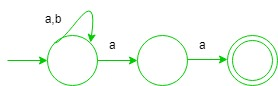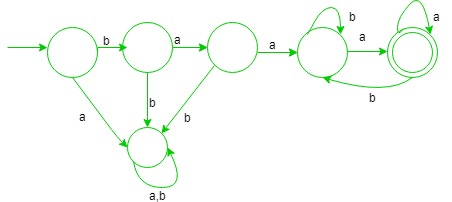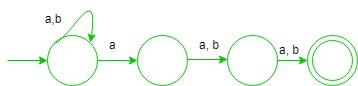GeeksforGeeks App
Open AppBrowser
Continue

These questions for practice purpose for GATE CS Exam. Ques-1: Which one of the following language is Regular? (A) {wxwR | w,x ∈ (a+b)+} (B) {wxwR | w ∈ (a+b)*, x ∈ {a,b}} (C) {wwRx | w,x ∈ (a+b)+} (D) {wwR | w ∈ (a+b)*} Explanation:

• (A) It is correct, since this language can form regular expression which is {{ a(a + b)+a } + {b(a + b)+b}}, i.e., start and end with same symbol.
• (B) It is deterministic context free language since, string before and after ‘x’ are same so, it is matched.
• (C) It cannot be regular since, wwR is done at first which requires comparison which cannot be done via finite automata.
• (D) It is also not regular since, comparison is required.

Option (A) is true. Ques-2: Let w be any string of length n in {a, b}*. Consider ‘L’ be the set of all strings ending with at least n a’s. What is the minimum number of states in non deterministic finite automata that accept ‘L’? (A) (n+3) (B) (n+1) (C) n (D) 2n Explanation: It is correct since, the minimum number of states required for NFA for ending with at least 2 a’s is (2 + 1) i.e., regular expression will be (a + b)*aaHence, Number of states required for at least n a’s will be (n+1). Option (B) is true. Ques-3: What is the minimum number of states in deterministic finite automata (DFA) for string starting with ba2 and ending with ‘a’ over alphabet {a, b}? (A) Ten (B) Nine (C) Eight (D) Six Explanation:In the above DFA, minimum number of states required is six. Option (D) is correct. Ques-4: Consider the following statements:

```S1 = {(an)m | n = 0}
S2 = {anbn | n>=1} U {anbm | n>=1, m>=1} ```

Which one of the following is regular? (A) only S1 (B) only S2 (C) both S1 and S2 (D) none Explanation: Both given languages are regular. Option (C) is correct. Ques-5: What is the number of states in minimal NFA(non deterministic finite automata), which accepts set of all strings in which the third last symbol is ‘a’ over alphabet {a, b}? (A) three (B) four (C) six (D) five Explanation:In the above NFA, minimum number of states required is four. Option (B) is true.

My Personal Notes arrow_drop_up